• Call Now

1800-102-2727•

# Transformer: Construction, Principle & Working, Efficiency

Have you ever wondered what a transformer is? Perhaps you must have seen a transformer installed in your localityThey are huge, bulky devices usually installed in an electrical substation. But what role does the transformer play in our electric network? In this article we will try to decode the transformer.

• Transformer
• Principle of transformer
• Working of transformer
• Power relations in transformer
• Efficiency of transformer
• Practice Problems
• FAQs

## Transformer

Transformer is a device used to increase or decrease alternating voltage by using mutual induction. One of the major advantages of AC over DC for electric power distribution is the ease with which voltage levels can be stepped up and down. For a long distance power transmission, it is desirable to use high voltage and as small a current as possible. Because this reduces the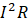losses in the transmission lines, and as smaller wires can be used, it saves material costs. Present-day transmission lines routinely operate at RMS voltage of the order of 500 kV. In generating equipment and residential and industrial power distribution, though, safety and insulation requirements necessitate relatively low voltages. The standard voltage for household wiring is 220 V in India and 120 V in the United States. The necessary voltage conservation is accomplished by the use of transformers.f

When an alternating voltage source is connected to a coil as shown above, it produces a changing magnetic field in the surrounding region of the coil. When another coil (known as ‘Secondary coil’) is brought in the vicinity of the primary coil with the help of iron core (as shown below), the phenomenon of mutual inductance is seen to happen between these coils and EMF is induced in the secondary coil. Hence, any electrical appliances can be connected at the terminal of the secondary coil.## Working of transformer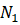and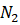are the number of turns in the primary and secondary winding, respectively. Varying the number of turns in the primary and secondary winding, we can produce a ‘Step up’ or ‘Step down’ transformer. The iron core is made up of ferromagnetic material because the ferromagnetic material generates a large magnetic field inside due to a small external magnetic field. The small magnetic field, which the primary winding generates, produces a large magnetic field inside the core and hence, the same magnetic flux is generated through each winding. Let that flux through each turn is 𝜙.

Applying KVL in the primary circuit (consists of AC source and the primary winding) in the clockwise direction, we get,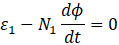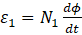………….(1)

Applying KVL in the secondary circuit in the clockwise direction, we get,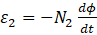………….(2)

Dividing equation (1) by (2) we get,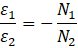The negative sign shows that the EMF induced in the secondary coil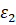is 180° out of phase with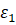.

Magnitude wise the above relation can be written as: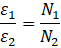## Power relations in transformer

The power delivered by the AC supply i .e. , the input power is,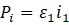The output power is given by,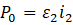Assuming power loss to be zero in the transformer, we can write the following by applying the principle of energy conservation.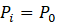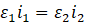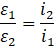Therefore, by combining the relationship written before with the relationship written above, we can write the following: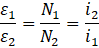## Efficiency of transformer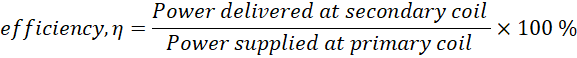If we denote power loss as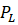, then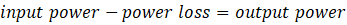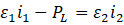Efficiency in an ideal case,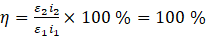, as in ideal caseEfficiency in real life scenarios,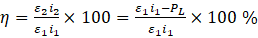## Practice Problems

Q. In a transformer, the output voltage is 20 𝑉. If the ratio of number of turns in the primary to secondary is 2: 1, what is the input voltage?

A. Given,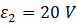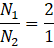we know that,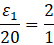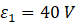Voltage on the input side is 40 V.

Q. In the previous question, if the current in secondary coil is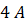. Then find the current in the primary coil?

A. Given,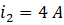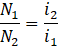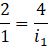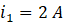The current in the primary coil will be 2 A.

Q. A transformer has 600 turns of the primary winding and 20 turns of the secondary winding. If the secondary circuit is open and the primary voltage is 140 V, calculate the secondary voltage?

A.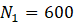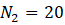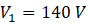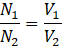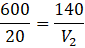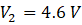Q. Input supply power of transformer is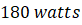and power lost in the transmission is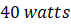. Then find the efficiency of the transformer?

A.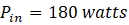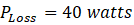We know,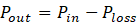Therefore,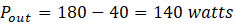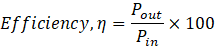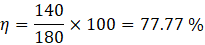## FAQs

Q. What is the efficiency of an ideal transformer?
A. There is no power loss in the ideal transformer so the efficiency is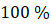.

Q. What is the working principle of a transformer?
A. Transformer works on the principle of mutual induction.

Q. Can a transformer work on DC voltage?
A. No, the transformer can not work on DC voltage. It works only on AC voltage using the principle of mutual induction.

Q. Is there any electrical wired connection between the input and output coil of a transformer?
A. There is no electrical wired connection between the input and output coil of the transformer but they are linked magnetically.Talk to our expert
Resend OTP Timer =
By submitting up, I agree to receive all the Whatsapp communication on my registered number and Aakash terms and conditions and privacy policy